# Chapter 7 Lossless Compression Algorithms 7 1 Introduction

• Slides: 49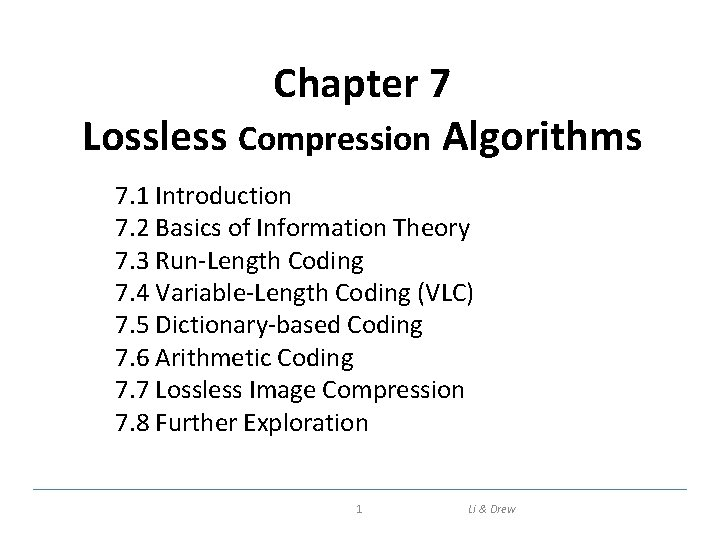Chapter 7 Lossless Compression Algorithms 7. 1 Introduction 7. 2 Basics of Information Theory 7. 3 Run-Length Coding 7. 4 Variable-Length Coding (VLC) 7. 5 Dictionary-based Coding 7. 6 Arithmetic Coding 7. 7 Lossless Image Compression 7. 8 Further Exploration 1 Li & Drew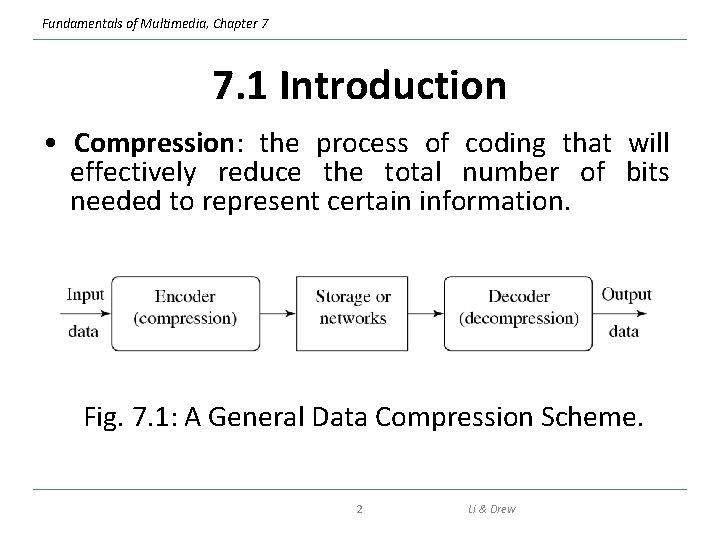Fundamentals of Multimedia, Chapter 7 7. 1 Introduction • Compression: the process of coding that will effectively reduce the total number of bits needed to represent certain information. Fig. 7. 1: A General Data Compression Scheme. 2 Li & DrewFundamentals of Multimedia, Chapter 7 Introduction (cont’d) • If the compression and decompression processes induce no information loss, then the compression scheme is lossless; otherwise, it is lossy. • Compression ratio: (7. 1) B 0 – number of bits before compression B 1 – number of bits after compression 3 Li & DrewFundamentals of Multimedia, Chapter 7 7. 2 Basics of Information Theory • The entropy η of an information source with alphabet S = {s 1, s 2, . . . , sn} is: (7. 2) (7. 3) pi – probability that symbol si will occur in S. – indicates the amount of information ( selfinformation as defined by Shannon) contained in si, which corresponds to the number of bits needed to encode si. 4 Li & Drew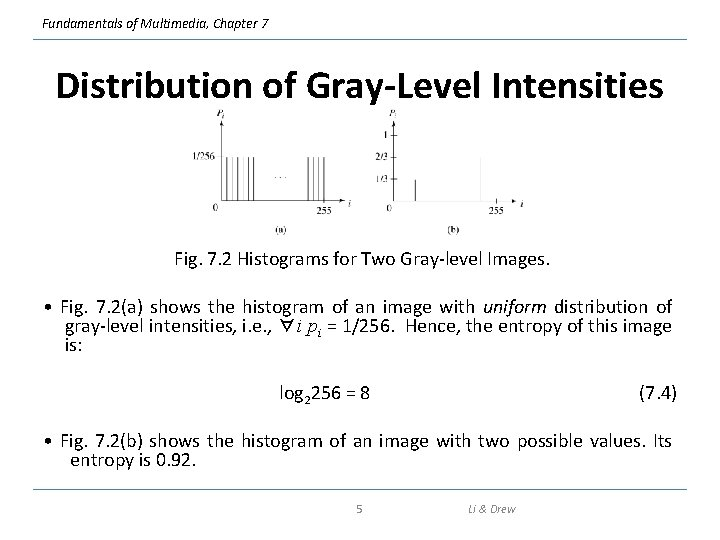Fundamentals of Multimedia, Chapter 7 Distribution of Gray-Level Intensities Fig. 7. 2 Histograms for Two Gray-level Images. • Fig. 7. 2(a) shows the histogram of an image with uniform distribution of gray-level intensities, i. e. , ∀i pi = 1/256. Hence, the entropy of this image is: log 2256 = 8 (7. 4) • Fig. 7. 2(b) shows the histogram of an image with two possible values. Its entropy is 0. 92. 5 Li & Drew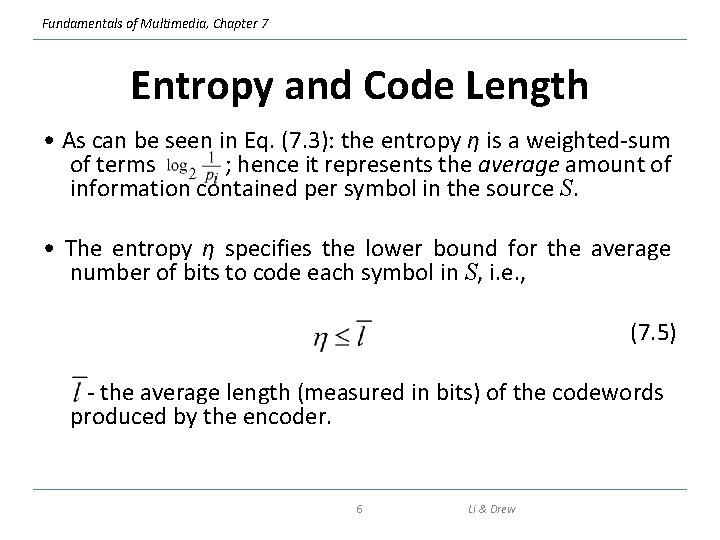Fundamentals of Multimedia, Chapter 7 Entropy and Code Length • As can be seen in Eq. (7. 3): the entropy η is a weighted-sum of terms ; hence it represents the average amount of information contained per symbol in the source S. • The entropy η specifies the lower bound for the average number of bits to code each symbol in S, i. e. , (7. 5) - the average length (measured in bits) of the codewords produced by the encoder. 6 Li & DrewFundamentals of Multimedia, Chapter 7 7. 3 Run-Length Coding • Memoryless Source: an information source that is independently distributed. Namely, the value of the current symbol does not depend on the values of the previously appeared symbols. • Instead of assuming memoryless source, Run-Length Coding (RLC) exploits memory present in the information source. • Rationale for RLC: if the information source has the property that symbols tend to form continuous groups, then such symbol and the length of the group can be coded. 7 Li & Drew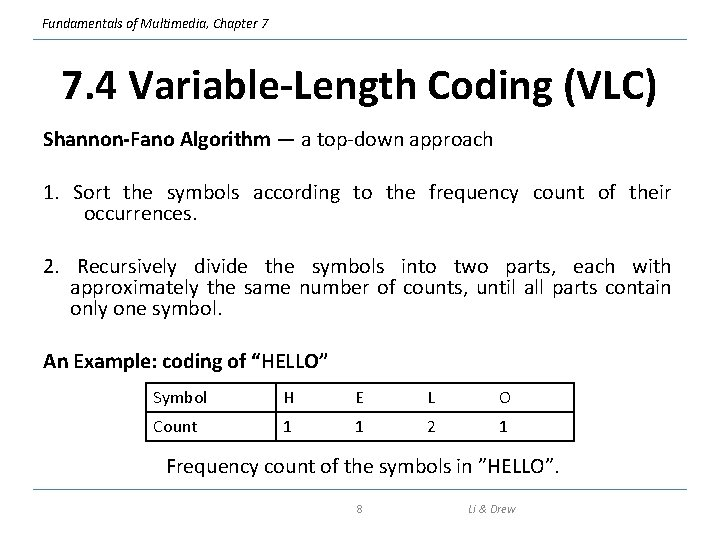Fundamentals of Multimedia, Chapter 7 7. 4 Variable-Length Coding (VLC) Shannon-Fano Algorithm — a top-down approach 1. Sort the symbols according to the frequency count of their occurrences. 2. Recursively divide the symbols into two parts, each with approximately the same number of counts, until all parts contain only one symbol. An Example: coding of “HELLO” Symbol H E L O Count 1 1 2 1 Frequency count of the symbols in ”HELLO”. 8 Li & DrewFundamentals of Multimedia, Chapter 7 Fig. 7. 3: Coding Tree for HELLO by Shannon-Fano. 9 Li & Drew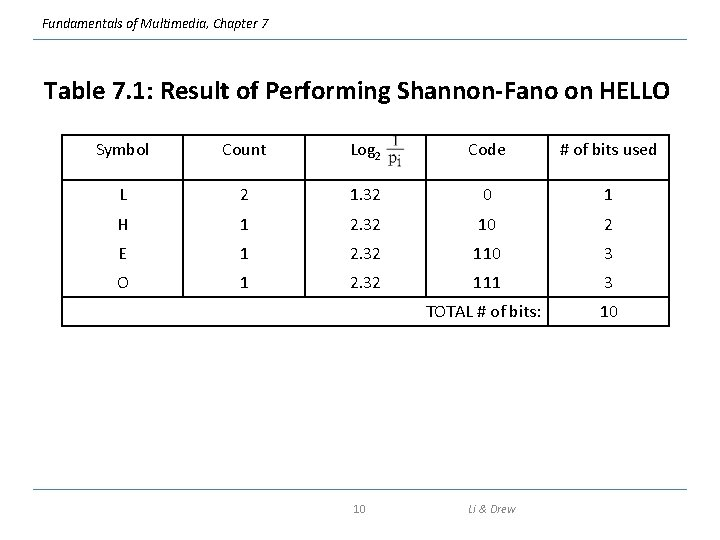Fundamentals of Multimedia, Chapter 7 Table 7. 1: Result of Performing Shannon-Fano on HELLO Symbol Count Log 2 Code # of bits used L 2 1. 32 0 1 H 1 2. 32 10 2 E 1 2. 32 110 3 O 1 2. 32 111 3 TOTAL # of bits: 10 10 Li & Drew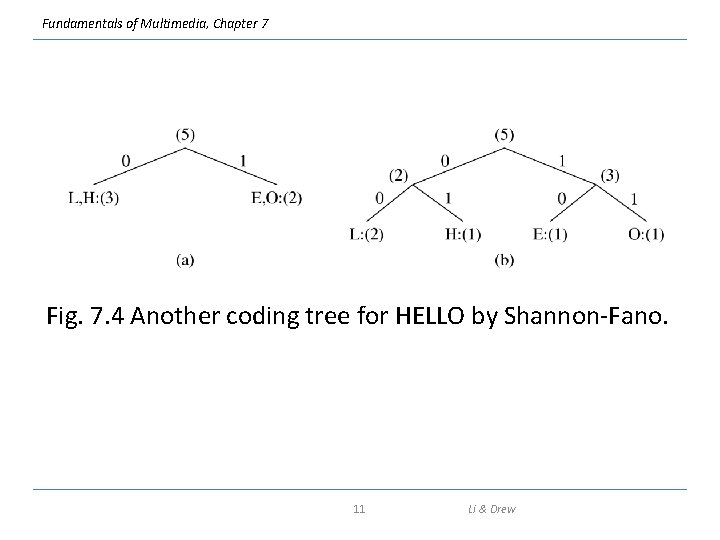Fundamentals of Multimedia, Chapter 7 Fig. 7. 4 Another coding tree for HELLO by Shannon-Fano. 11 Li & Drew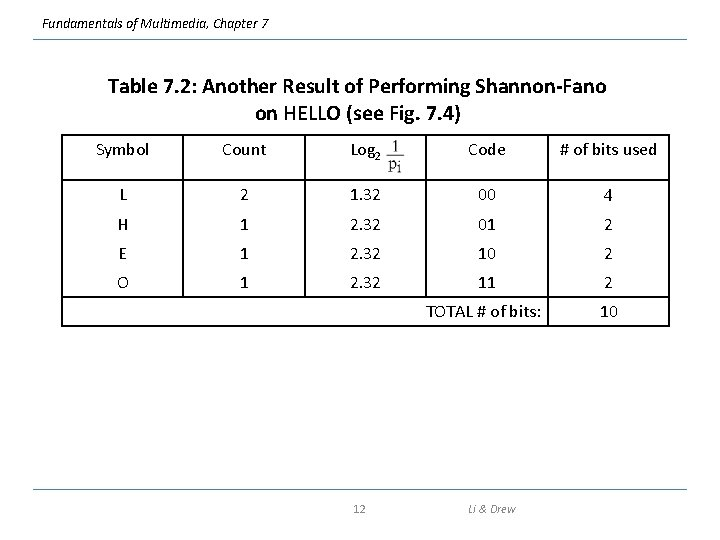Fundamentals of Multimedia, Chapter 7 Table 7. 2: Another Result of Performing Shannon-Fano on HELLO (see Fig. 7. 4) Symbol Count Log 2 Code # of bits used L 2 1. 32 00 4 H 1 2. 32 01 2 E 1 2. 32 10 2 O 1 2. 32 11 2 TOTAL # of bits: 10 12 Li & Drew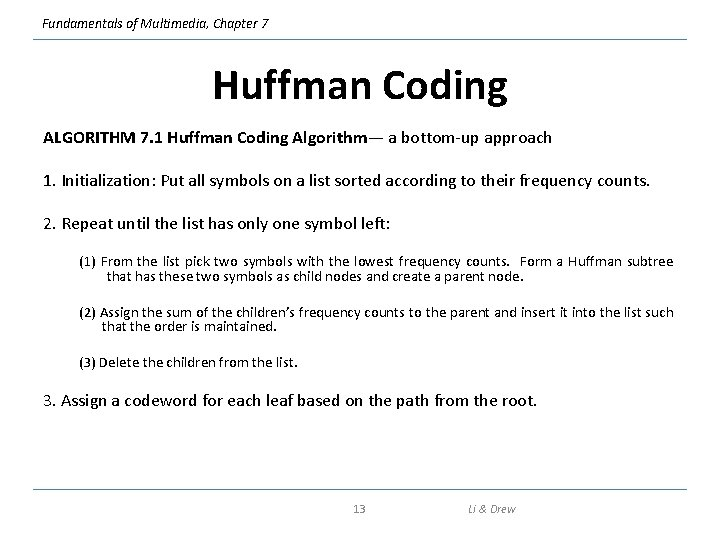Fundamentals of Multimedia, Chapter 7 Huffman Coding ALGORITHM 7. 1 Huffman Coding Algorithm— a bottom-up approach 1. Initialization: Put all symbols on a list sorted according to their frequency counts. 2. Repeat until the list has only one symbol left: (1) From the list pick two symbols with the lowest frequency counts. Form a Huffman subtree that has these two symbols as child nodes and create a parent node. (2) Assign the sum of the children’s frequency counts to the parent and insert it into the list such that the order is maintained. (3) Delete the children from the list. 3. Assign a codeword for each leaf based on the path from the root. 13 Li & Drew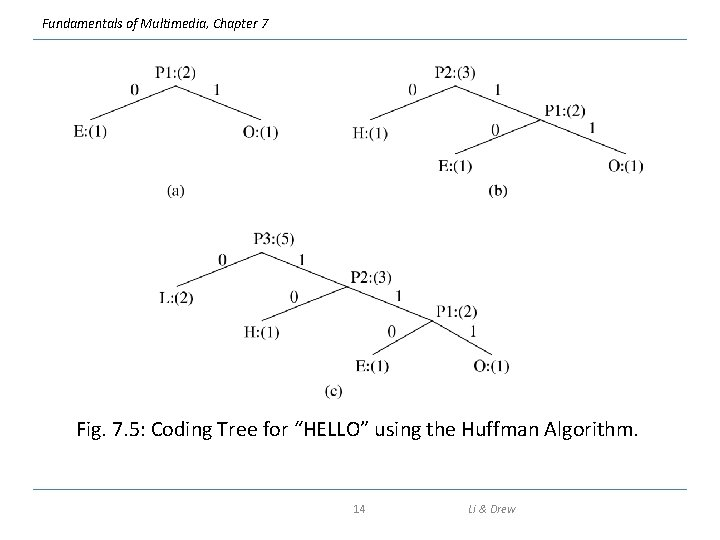Fundamentals of Multimedia, Chapter 7 Fig. 7. 5: Coding Tree for “HELLO” using the Huffman Algorithm. 14 Li & DrewFundamentals of Multimedia, Chapter 7 Huffman Coding (cont’d) In Fig. 7. 5, new symbols P 1, P 2, P 3 are created to refer to the parent nodes in the Huffman coding tree. The contents in the list are illustrated below: After initialization: After iteration (a): After iteration (b): After iteration (c): L L L P 3 15 H P 1 P 2 E H Li & Drew O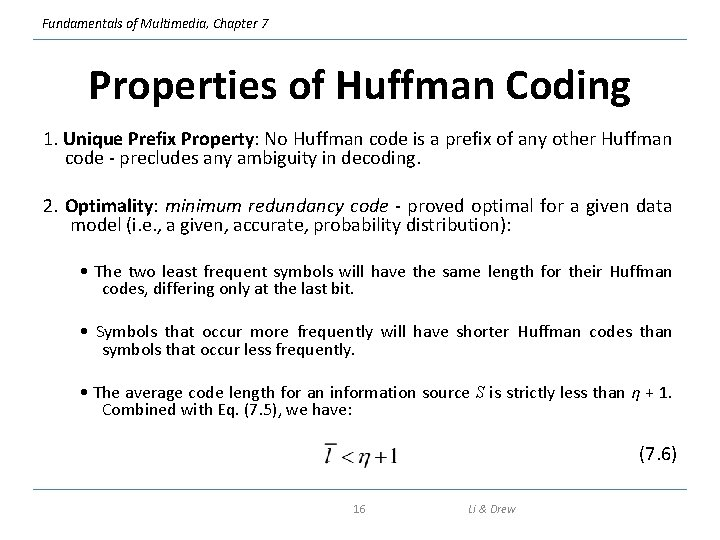Fundamentals of Multimedia, Chapter 7 Properties of Huffman Coding 1. Unique Prefix Property: No Huffman code is a prefix of any other Huffman code - precludes any ambiguity in decoding. 2. Optimality: minimum redundancy code - proved optimal for a given data model (i. e. , a given, accurate, probability distribution): • The two least frequent symbols will have the same length for their Huffman codes, differing only at the last bit. • Symbols that occur more frequently will have shorter Huffman codes than symbols that occur less frequently. • The average code length for an information source S is strictly less than η + 1. Combined with Eq. (7. 5), we have: (7. 6) 16 Li & Drew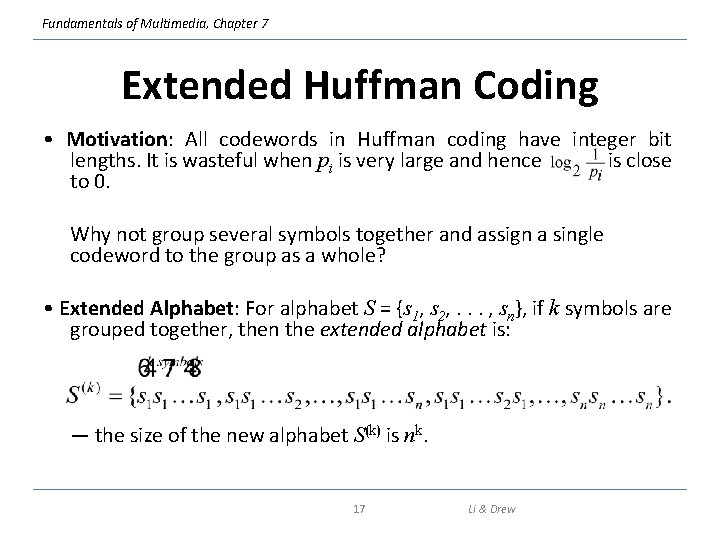Fundamentals of Multimedia, Chapter 7 Extended Huffman Coding • Motivation: All codewords in Huffman coding have integer bit lengths. It is wasteful when pi is very large and hence is close to 0. Why not group several symbols together and assign a single codeword to the group as a whole? • Extended Alphabet: For alphabet S = {s 1, s 2, . . . , sn}, if k symbols are grouped together, then the extended alphabet is: — the size of the new alphabet S(k) is nk. 17 Li & Drew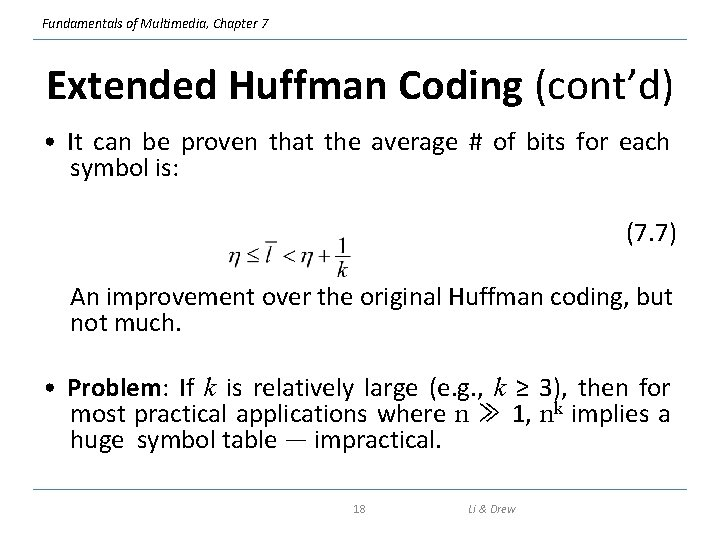Fundamentals of Multimedia, Chapter 7 Extended Huffman Coding (cont’d) • It can be proven that the average # of bits for each symbol is: (7. 7) An improvement over the original Huffman coding, but not much. • Problem: If k is relatively large (e. g. , k ≥ 3), then for most practical applications where n ≫ 1, nk implies a huge symbol table — impractical. 18 Li & Drew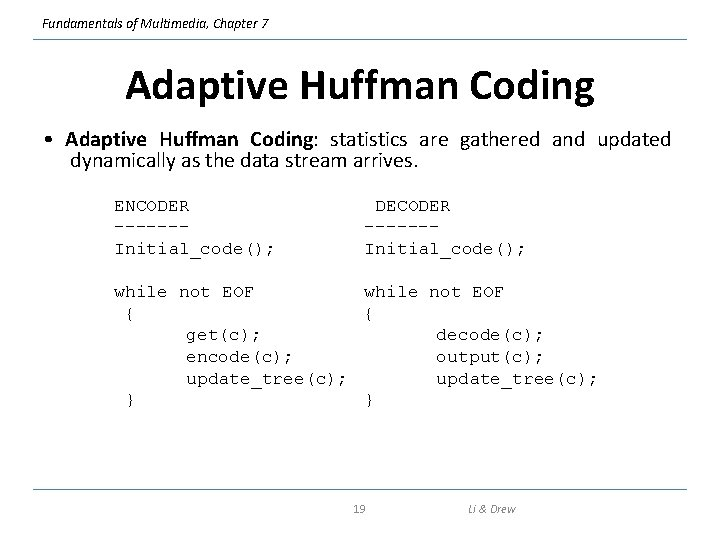Fundamentals of Multimedia, Chapter 7 Adaptive Huffman Coding • Adaptive Huffman Coding: statistics are gathered and updated dynamically as the data stream arrives. ENCODER ------Initial_code(); DECODER ------Initial_code(); while not EOF { { get(c); decode(c); encode(c); output(c); update_tree(c); } } 19 Li & Drew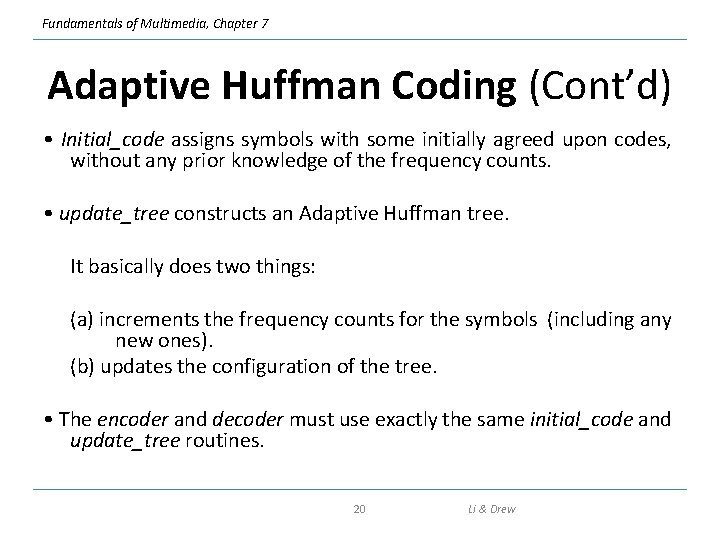Fundamentals of Multimedia, Chapter 7 Adaptive Huffman Coding (Cont’d) • Initial_code assigns symbols with some initially agreed upon codes, without any prior knowledge of the frequency counts. • update_tree constructs an Adaptive Huffman tree. It basically does two things: (a) increments the frequency counts for the symbols (including any new ones). (b) updates the configuration of the tree. • The encoder and decoder must use exactly the same initial_code and update_tree routines. 20 Li & DrewFundamentals of Multimedia, Chapter 7 Notes on Adaptive Huffman Tree Updating • Nodes are numbered in order from left to right, bottom to top. The numbers in parentheses indicates the count. • The tree must always maintain its sibling property, i. e. , all nodes (internal and leaf) are arranged in the order of increasing counts. If the sibling property is about to be violated, a swap procedure is invoked to update the tree by rearranging the nodes. • When a swap is necessary, the farthest node with count N is swapped with the node whose count has just been increased to N +1. 21 Li & DrewFundamentals of Multimedia, Chapter 7 Fig. 7. 6: Node Swapping for Updating an Adaptive Huffman Tree 22 Li & Drew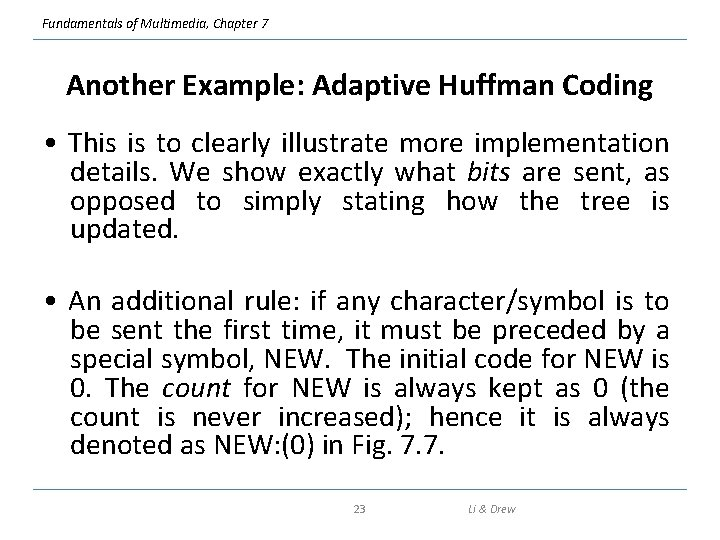Fundamentals of Multimedia, Chapter 7 Another Example: Adaptive Huffman Coding • This is to clearly illustrate more implementation details. We show exactly what bits are sent, as opposed to simply stating how the tree is updated. • An additional rule: if any character/symbol is to be sent the first time, it must be preceded by a special symbol, NEW. The initial code for NEW is 0. The count for NEW is always kept as 0 (the count is never increased); hence it is always denoted as NEW: (0) in Fig. 7. 7. 23 Li & DrewFundamentals of Multimedia, Chapter 7 Table 7. 3: Initial code assignment for AADCCDD using adaptive Huffman coding. Initial Code ----------NEW: 0 A: 00001 B: 00010 C: 00011 D: 00100. . . 24 Li & DrewFundamentals of Multimedia, Chapter 7 Fig. 7. 7 Adaptive Huffman tree for AADCCDD. 25 Li & DrewFundamentals of Multimedia, Chapter 7 Fig. 7. 7 (cont’d) Adaptive Huffman tree for AADCCDD. 26 Li & Drew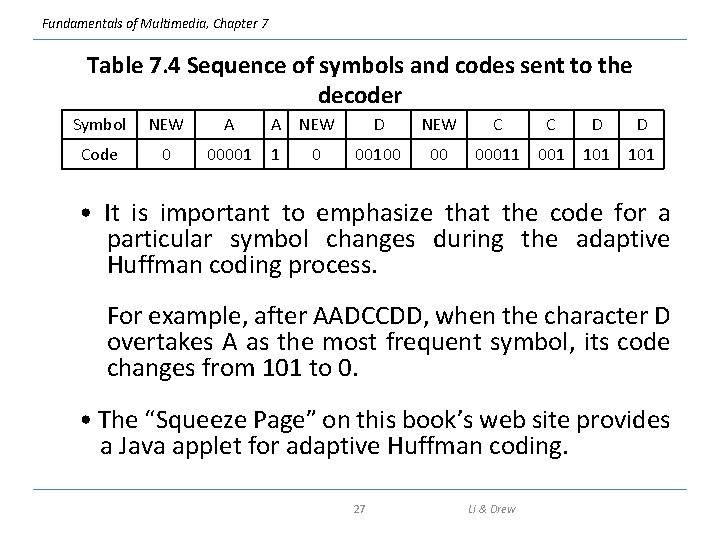Fundamentals of Multimedia, Chapter 7 Table 7. 4 Sequence of symbols and codes sent to the decoder Symbol NEW A Code 0 00001 A NEW 1 0 D NEW 00100 00 C C D D 00011 001 101 • It is important to emphasize that the code for a particular symbol changes during the adaptive Huffman coding process. For example, after AADCCDD, when the character D overtakes A as the most frequent symbol, its code changes from 101 to 0. • The “Squeeze Page” on this book’s web site provides a Java applet for adaptive Huffman coding. 27 Li & DrewFundamentals of Multimedia, Chapter 7 7. 5 Dictionary-based Coding • LZW uses fixed-length codewords to represent variablelength strings of symbols/characters that commonly occur together, e. g. , words in English text. • the LZW encoder and decoder build up the same dictionary dynamically while receiving the data. • LZW places longer and longer repeated entries into a dictionary, and then emits the code for an element, rather than the string itself, if the element has already been placed in the dictionary. 28 Li & DrewFundamentals of Multimedia, Chapter 7 ALGORITHM 7. 2 - LZW Compression BEGIN s = next input character; while not EOF { c = next input character; if s + c exists in the dictionary s = s + c; else { output the code for s; add string s + c to the dictionary with a new code; s = c; } } output the code for s; END 29 Li & DrewFundamentals of Multimedia, Chapter 7 Example 7. 2 LZW compression for string “ABABBABCABABBA” • Let’s start with a very simple dictionary (also referred to as a “string table”), initially containing only 3 characters, with codes as follows: Code String 1 A 2 B 3 C • Now if the input string is “ABABBABCABABBA”, the LZW compression algorithm works as follows: 30 Li & DrewFundamentals of Multimedia, Chapter 7 S A B A AB B BA B C A AB ABB A C B A B C A B B A EOF Output Code String 1 2 3 A B C 1 2 4 5 AB BA 4 6 ABB 5 2 3 7 8 9 BAB BC CA 4 10 ABA 6 1 11 ABBA • The output codes are: 1 2 4 5 2 3 4 6 1. Instead of sending 14 characters, only 9 codes need to be sent (compression ratio = 14/9 = 1. 56). 31 Li & Drew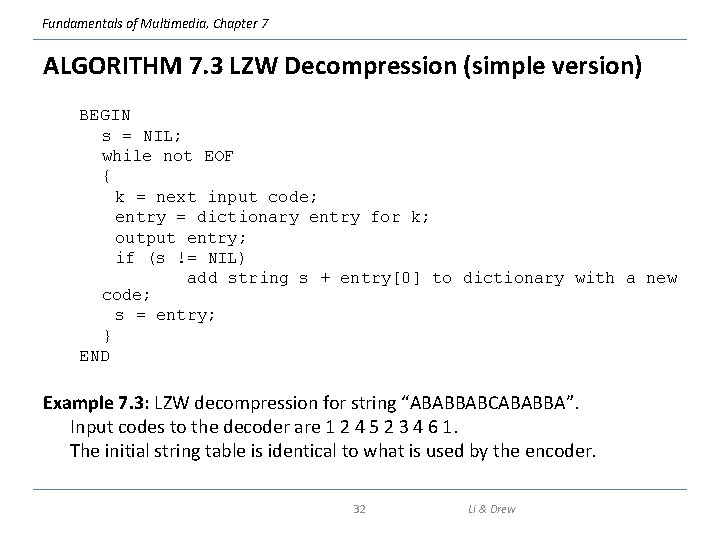Fundamentals of Multimedia, Chapter 7 ALGORITHM 7. 3 LZW Decompression (simple version) BEGIN s = NIL; while not EOF { k = next input code; entry = dictionary entry for k; output entry; if (s != NIL) add string s + entry to dictionary with a new code; s = entry; } END Example 7. 3: LZW decompression for string “ABABBABCABABBA”. Input codes to the decoder are 1 2 4 5 2 3 4 6 1. The initial string table is identical to what is used by the encoder. 32 Li & Drew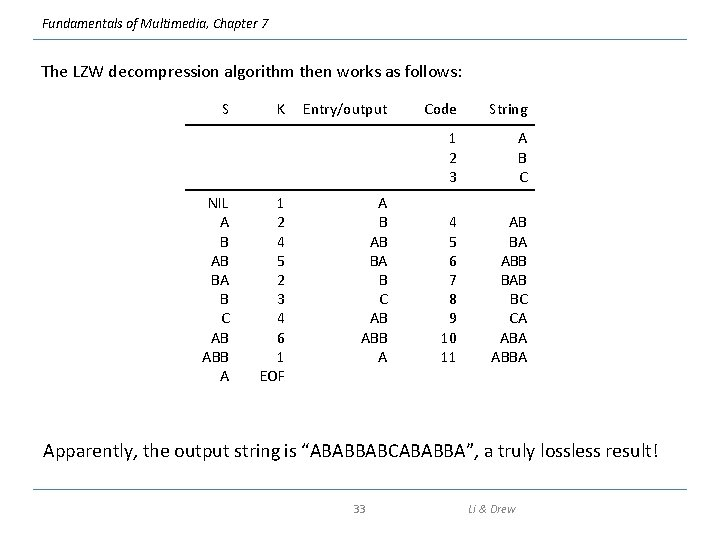Fundamentals of Multimedia, Chapter 7 The LZW decompression algorithm then works as follows: S NIL A B AB BA B C AB ABB A K 1 2 4 5 2 3 4 6 1 EOF Entry/output A B AB BA B C AB ABB A Code String 1 2 3 A B C 4 5 6 7 8 9 10 11 AB BA ABB BAB BC CA ABBA Apparently, the output string is “ABABBABCABABBA”, a truly lossless result! 33 Li & Drew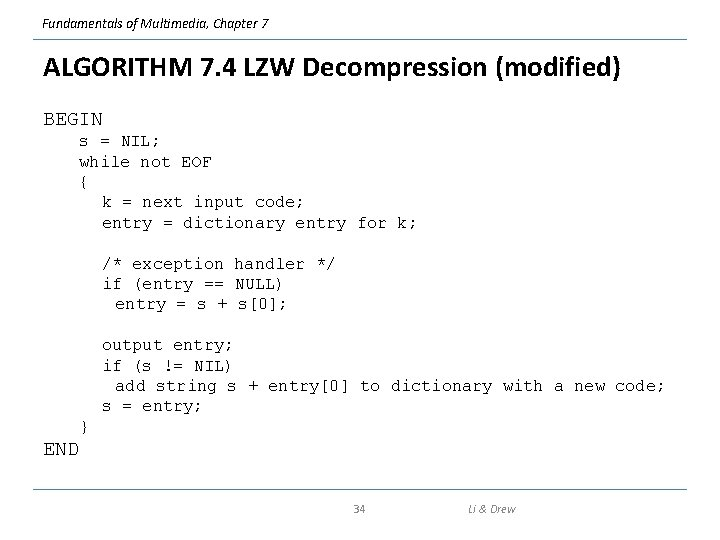Fundamentals of Multimedia, Chapter 7 ALGORITHM 7. 4 LZW Decompression (modified) BEGIN s = NIL; while not EOF { k = next input code; entry = dictionary entry for k; /* exception handler */ if (entry == NULL) entry = s + s; output entry; if (s != NIL) add string s + entry to dictionary with a new code; s = entry; } END 34 Li & DrewFundamentals of Multimedia, Chapter 7 LZW Coding (cont’d) • In real applications, the code length l is kept in the range of [l 0, lmax]. The dictionary initially has a size of 2 l 0. When it is filled up, the code length will be increased by 1; this is allowed to repeat until l = lmax. • When lmax is reached and the dictionary is filled up, it needs to be flushed (as in Unix compress, or to have the LRU (least recently used) entries removed. 35 Li & DrewFundamentals of Multimedia, Chapter 7 7. 6 Arithmetic Coding • Arithmetic coding is a more modern coding method that usually out-performs Huffman coding. • Huffman coding assigns each symbol a codeword which has an integral bit length. Arithmetic coding can treat the whole message as one unit. • A message is represented by a half-open interval [a, b) where a and b are real numbers between 0 and 1. Initially, the interval is [0, 1). When the message becomes longer, the length of the interval shortens and the number of bits needed to represent the interval increases. 36 Li & Drew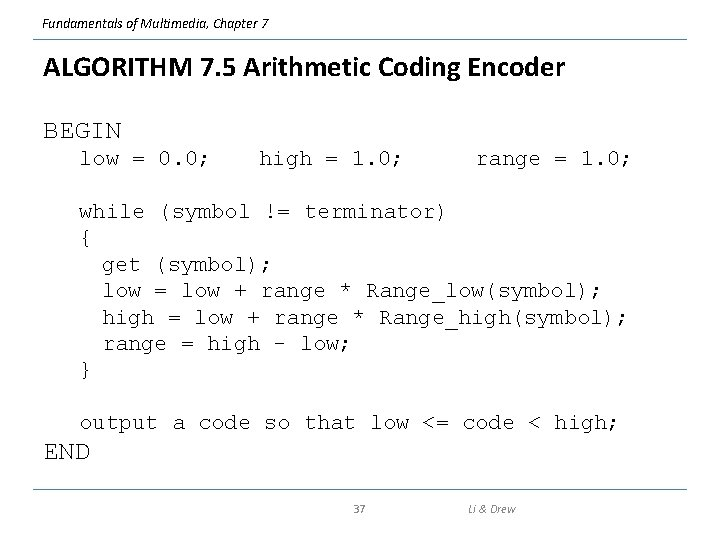Fundamentals of Multimedia, Chapter 7 ALGORITHM 7. 5 Arithmetic Coding Encoder BEGIN low = 0. 0; high = 1. 0; range = 1. 0; while (symbol != terminator) { get (symbol); low = low + range * Range_low(symbol); high = low + range * Range_high(symbol); range = high - low; } output a code so that low <= code < high; END 37 Li & DrewFundamentals of Multimedia, Chapter 7 Example: Encoding in Arithmetic Coding Symbol Probability Range A 0. 2 [0, 0. 2) B 0. 1 [0. 2, 0. 3) C 0. 2 [0. 3, 0. 5) D 0. 05 [0. 5, 0. 55) E 0. 3 [0. 55, 0. 85) F 0. 05 [0. 85, 0. 9) G 0. 1 [0. 9, 1. 0) (a) Probability distribution of symbols. Fig. 7. 8: Arithmetic Coding: Encode Symbols “CAEE\$” 38 Li & Drew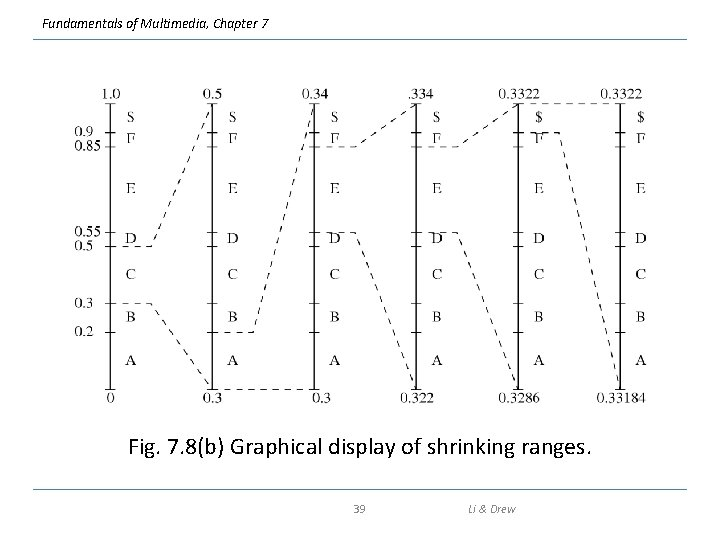Fundamentals of Multimedia, Chapter 7 Fig. 7. 8(b) Graphical display of shrinking ranges. 39 Li & DrewFundamentals of Multimedia, Chapter 7 Example: Encoding in Arithmetic Coding Symbol Low High Range 0 1. 0 C 0. 3 0. 5 0. 2 A 0. 30 0. 34 0. 04 E 0. 322 0. 334 0. 012 E 0. 3286 0. 3322 0. 0036 \$ 0. 33184 0. 33220 0. 00036 (c) New low, high, and range generated. Fig. 7. 8 (cont’d): Arithmetic Coding: Encode Symbols “CAEE\$” 40 Li & Drew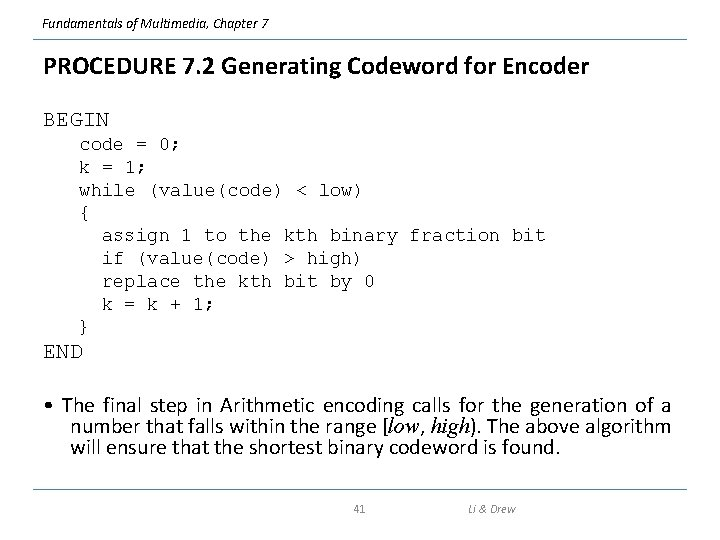Fundamentals of Multimedia, Chapter 7 PROCEDURE 7. 2 Generating Codeword for Encoder BEGIN code = 0; k = 1; while (value(code) < low) { assign 1 to the kth binary fraction bit if (value(code) > high) replace the kth bit by 0 k = k + 1; } END • The final step in Arithmetic encoding calls for the generation of a number that falls within the range [low, high). The above algorithm will ensure that the shortest binary codeword is found. 41 Li & Drew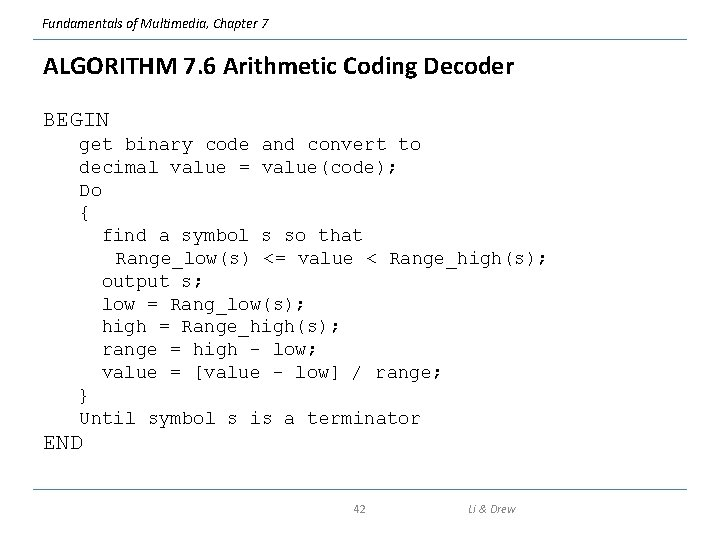Fundamentals of Multimedia, Chapter 7 ALGORITHM 7. 6 Arithmetic Coding Decoder BEGIN get binary code and convert to decimal value = value(code); Do { find a symbol s so that Range_low(s) <= value < Range_high(s); output s; low = Rang_low(s); high = Range_high(s); range = high - low; value = [value - low] / range; } Until symbol s is a terminator END 42 Li & Drew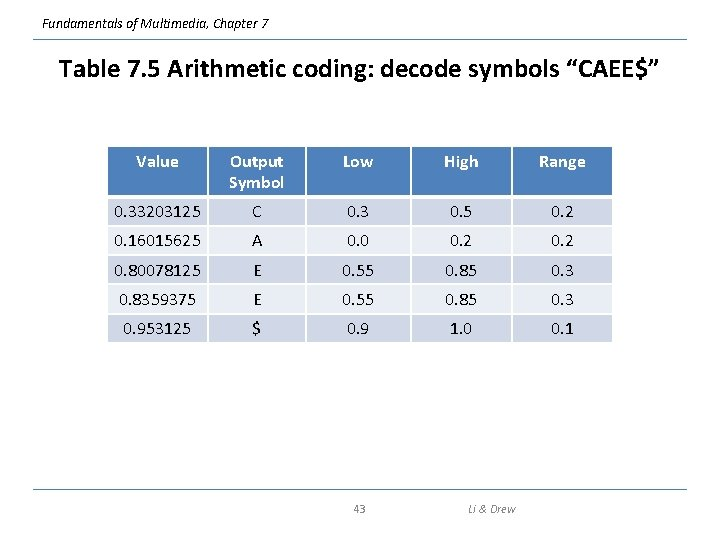Fundamentals of Multimedia, Chapter 7 Table 7. 5 Arithmetic coding: decode symbols “CAEE\$” Value Output Symbol Low High Range 0. 33203125 C 0. 3 0. 5 0. 2 0. 16015625 A 0. 0 0. 2 0. 80078125 E 0. 55 0. 85 0. 3 0. 8359375 E 0. 55 0. 85 0. 3 0. 953125 \$ 0. 9 1. 0 0. 1 43 Li & DrewFundamentals of Multimedia, Chapter 7 7. 7 Lossless Image Compression • Approaches of Differential Coding of Images: – Given an original image I(x, y), using a simple difference operator we can define a difference image d(x, y) as follows: d(x, y) = I(x, y) − I(x − 1, y) (7. 9) or use the discrete version of the 2 -D Laplacian operator to define a difference image d(x, y) as d(x, y) = 4 I(x, y) − I(x, y − 1) − I(x, y +1) − I(x+1, y) − I(x − 1, y) (7. 10) • Due to spatial redundancy existed in normal images I, the difference image d will have a narrower histogram and hence a smaller entropy, as shown in Fig. 7. 9. 44 Li & Drew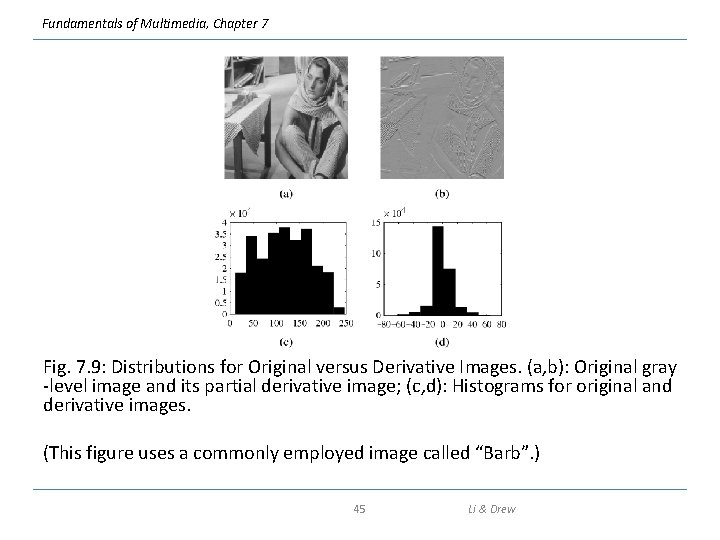Fundamentals of Multimedia, Chapter 7 Fig. 7. 9: Distributions for Original versus Derivative Images. (a, b): Original gray -level image and its partial derivative image; (c, d): Histograms for original and derivative images. (This figure uses a commonly employed image called “Barb”. ) 45 Li & Drew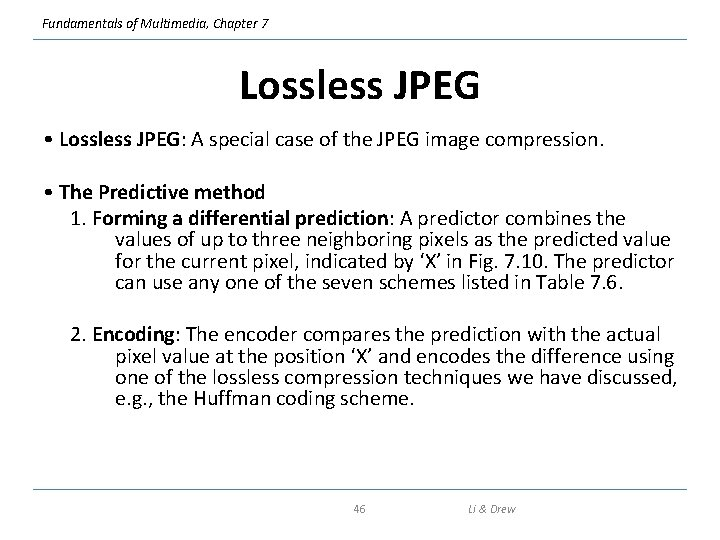Fundamentals of Multimedia, Chapter 7 Lossless JPEG • Lossless JPEG: A special case of the JPEG image compression. • The Predictive method 1. Forming a differential prediction: A predictor combines the values of up to three neighboring pixels as the predicted value for the current pixel, indicated by ‘X’ in Fig. 7. 10. The predictor can use any one of the seven schemes listed in Table 7. 6. 2. Encoding: The encoder compares the prediction with the actual pixel value at the position ‘X’ and encodes the difference using one of the lossless compression techniques we have discussed, e. g. , the Huffman coding scheme. 46 Li & DrewFundamentals of Multimedia, Chapter 7 Fig. 7. 10: Neighboring Pixels for Predictors in Lossless JPEG. • Note: Any of A, B, or C has already been decoded before it is used in the predictor, on the decoder side of an encode-decode cycle. 47 Li & DrewFundamentals of Multimedia, Chapter 7 Table 7. 6: Predictors for Lossless JPEG Predictor Prediction P 1 A P 2 B P 3 C P 4 A+B–C P 5 A + (B – C) / 2 P 6 B + (A – C) / 2 P 7 (A + B) / 2 48 Li & Drew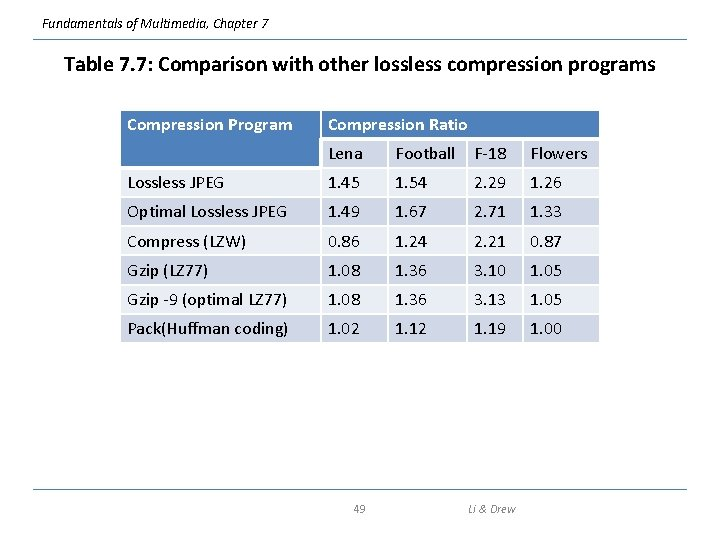Fundamentals of Multimedia, Chapter 7 Table 7. 7: Comparison with other lossless compression programs Compression Program Compression Ratio Lena Football F-18 Flowers Lossless JPEG 1. 45 1. 54 2. 29 1. 26 Optimal Lossless JPEG 1. 49 1. 67 2. 71 1. 33 Compress (LZW) 0. 86 1. 24 2. 21 0. 87 Gzip (LZ 77) 1. 08 1. 36 3. 10 1. 05 Gzip -9 (optimal LZ 77) 1. 08 1. 36 3. 13 1. 05 Pack(Huffman coding) 1. 02 1. 19 1. 00 49 Li & Drew